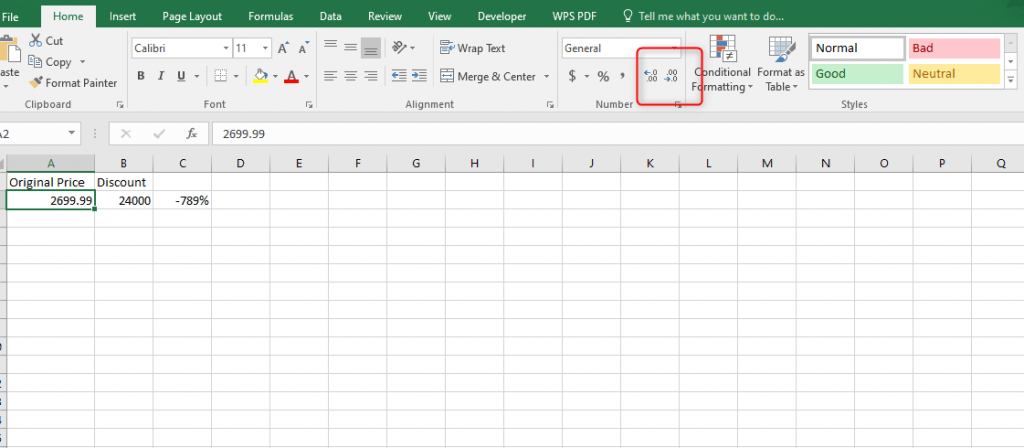# How to calculate discount in Excel

Discount is the amount deducted from the regular price of a commodity. This is usually found by deducting the selling price from the regular or marked price of a commodity. Discount can also be expressed in percentage by taking the regular price multiplied by the rate of discount.

## Example

A DVD player costs 27000. But when it is sold at a discount of 3000 is given to the customer. The Cost at which the DVD player bought = Original cost of a DVD player – Discount offered

= 27000 – 3000 = 24000

So the price of the DVD after the discount is 24000

When the original price and discount of the item is known % discount can be calculated. On the other hand, if you have the original price and the % discount you can calculate the discount. Having known the basic principle of discounting it is important to understand how to find the discount and percentage discount in excel.

## How to calculate the percentage Discount

If you have the original price and price that is discount what you do is;

1. First, take the discount price and divide it by the original price. In our example =B2/A2The cost of the DVD will be 24000 which is 89% of the original price

2. The next thing to do is to subtract 1 from what you have gotten.If you are paying 89% of the original price you will have gotten a percentage discount of 11%

3. To change the value to percentage go to the home tab, on the category of numbers click the percentage symbol to convert the decimal to percentage.This will convert the result into a percentage discount.## How to calculate the Discount price

If you are given the original price and the discount in percentage, it is possible to calculate the price as follows;

1. The first thing you will have to do is subtract the percentage discount given from 1.This implies that you will be required to pay 89% of the original price.

2. Take the 89% and multiply it with the original price to find the discount.The DVD will still be 89% of the original price which is 24000.

## How to calculate the original price

When you have the discount and the percentage discount you can be able to calculate the original price of the product. We saw that the discounted price was a product of the original price and (1- % Discount). To find the original price;

1. We simply divide the price of discount by (1 – % Discount)The deviation that we have from the original price is a decimal problem. To manage decimal numbers in your results.

2. Put the cursor and select the cell with the decimals3. Go to the home tab.

4. Use the options increase/ decrease decimals and choose as you desire.Alternatively,

1. Right-click in the cell containing the decimals

2. Choose the option format cells after which a screen will pop up, then the number tab.3. On the catalog, click on Number and change the decimal as you desire.